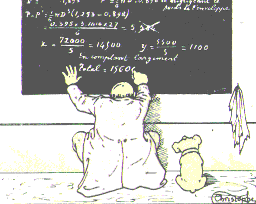# Month: November 2018

Here are the Mathematical Citation Quotient (MCQ) for journals in probability, statistics, analysis, and general mathematics. The numbers were obtained using a home brewed Python script extracting data from MathSciNet. The graphics were obtained by using LibreOffice.

Recall that the MCQ is a ratio of two counts for a selected journal and a selected year.  The MCQ for year $Y$ and journal $J$ is given by the formula $\mathrm{MCQ}=m/n$ where

• $m$ is the total number of citations of papers published in jounal $J$ in years $Y-1$,…,$Y-5$ by papers published in year $Y$ in any journal known by MathSciNet;
• $n$ is the total number of papers published in journal $J$ in years $Y-1$,…,$Y-5$.

The Mathematical Reviews compute every year the MCQ for every indexed journal, and make it available on MathSciNet. This formula is very similar to the one of the five years impact factor, the main difference being the population of journals which is specifically mathematical for the MCQ (reference list journals) and the way the citations are extracted. Both biases are negative.Let $X={(X_t)}_{t\geq0}$ be an irreducible Markov process with generator $G$ and unique invariant law $\mu$. What is the difference between irreversible and out of equilibrium? If you do not know what is a generator, they you may replace it by a transition kernel without loss of interpretation.

Trajectories. A Markov process is a way to transform an initial law into a law of trajectory, by mean of a generator. The law of the trajectory ${(X_t)}_{t\geq0}$ depends on the law of $X_0$ as well as on the generator $G$. The equilibrium or invariant law $\mu$ depends only on $G$.

Reversibility. We say that $X$ is reversible when $X_0\sim\mu$ implies that for all $t\geq0$ the trajectory $(X_s)_{0\leq s\leq t}$  and the reversed trajectory ${(X_{t-s})}_{0\leq s\leq t}$ have same law. This means that if we start the process from its equilibrium, the notion of orientation of time is not visible. Up to regularity considerations, this is equivalent to say that the generator is a symmetric operator in $L^2(\mu)$. When $X$ is a finite Markov chain, this corresponds to the detailed balance condition $\mu(x)G(x,y)=\mu(y)G(y,x)$ for all $x,y$ in the state space. A famous theorem by Kolmogorov states that this is equivalent to say that the weight of all cycles with respect to the transition kernel or generator does not depend on the orientation of the cycle, and the remarkable fact about this criterion is that it does not involve $\mu$.

Out of equilibrium. We say that the process $X$ is out of equilibrium when it is not started from the equilibrium $\mu$ meaning that $X_0\not\sim\mu$. Up to regularity assumptions, for all initial law, the process converges in law to its equilibrium in long time, in other words $\lim_{t\to\infty}X_t=\mu$ in law. On the contrary, if $X_0\sim\mu$, then the process satisfies $X_t\sim\mu$ for all $t\geq0$ since $\mu$ is invariant, and we say in this case that the process is at equilibrium.

Conclusion. Being out of equilibrium or not has nothing to do with being reversible or not. We may start a reversible process or not, out of equilibrium, or not. If we start a reversible process out of equilibrium, then typically it will converge exponentially fast to its equilibrium $\mu$ in $L^2(\mu)$, and the exponential speed of this convergence is given by the spectral gap of $G$.This tiny blog-post is about almost sure convergence in probability theory. What are the great providers of almost sure convergence? The most natural answer is the Borel-Cantelli lemmas, the strong laws of large numbers, and the martingale convergence theorems. After years, I think that a great provider of almost sure convergence is the following:

• if $X$ is a random variable taking values in $[0,+\infty]$ and such that $\mathbb{E}(X)<\infty$ then $$X<\infty\text{ almost surely.}$$

This elementary fact…

• can be used to prove the first Borel-Cantelli lemma. Namely, if $(A_n)_n$ is a sequence of events such that $\sum_n\mathbb{P}(A_n)<\infty$ then this means by monotone convergence that $\mathbb{E}(\sum_n\mathbf{1}_{A_n})<\infty$ and thus $\sum_n\mathbf{1}_{A_n}<\infty$ almost surely, which means that $\mathbb{P}(\varliminf A_n^c)=1$, in other words $\mathbb{P}(\varlimsup A_n)=0$.
• can be used to prove the strong law of large numbers for independent random variables bounded in $\mathrm{L}^4$. Namely if ${(X_n)}_n$ are independent centered random variables bounded in $\mathrm{L}^4$ then, setting $S_n=\frac{1}{n}(X_1+\cdots+X_n)$, we have $\mathbb{E}(S_n^4)=\mathcal{O}(n^{-2})$, and thus $\sum_n\mathbb{E}(S_n^4)<\infty$. By monotone convergence this gives $\mathbb{E}(\sum_nS_n^4)<\infty$, and thus, almost surely, $\sum_n S_n^4<\infty$, which implies that almost surely $\lim_{n\to\infty}S_n=0$.
• can be placed at the heart of the proof via upcrossing of the Doob theorem of almost sure convergence of martingales bounded in $\mathrm{L}^1$. Idem for the theorem of convergence of martingales bounded in $\mathrm{L}^2$. The other martingale convergence theorems are corollaries.

Is it true that almost all almost sure statements in probability theory can be deduced from this elementary fact, directly or via its above mentioned consequences? Almost. Note however that the Strassen law of the iterated logarithm relies on both the first and second Borel-Cantelli lemmas. Another basic provider of almost sure convergence is the sigma-additivity of measures which gives that an at most countable intersection of almost sure events is almost sure.This image is taken from a Current Biology recent paper, see below. The original image comes form Google Earth and each black dot marks a small mound created by termites in a region of Brazil. This resembles amazingly to a determinantal point process, such as the complex Ginibre ensemble and its circular law. Collective phenomenon and self-organized structure…

Syntax · Style · .